# What is the inverse Pythagoras theorem?

It is already late in the evening, actually it is past midnight so I will keep the text of this post short. It was a nice day today and this evening I brewed the 23-th batch of a beer known as ‘Spin half beer’. (I name it that way because it contains only half of the dark malts I use in the beer known as dark matter…;) so it has nothing to do with electrons).

This is a very basic post about some ‘inverse Pythagoras theorem’ as came flying by in some math video. I was rather surprised that I have not seen it before but there are so many theorems out there using that old fashioned Euclidian geometry that I might have forgetten all about it.

Within 10 minutes I had a good proof for the 2D version of this ‘inverse Pythagoras theorem’. You can find it in the first picture below.

One day later when I was riding a bit around I tried to find the higher dimensional analog of that easy to understand 2D statement or theorem. And as such it crossed my mind the important role a distance number d played in my proof for the general theorem of Pythagoras that acts on simplexes that are the higer dimensional analog of 2D triangles.

Coming home it was easy to write out the details, but for me it was all so simple that does this stuff deserve the title ‘theorem’? Well make up your own mind about that, but if it is not a real complicated theorem it is still a nice and cute result…

This post is six pictures long (all 550×775 pixels beside the last one that needed a bit expansion because the math did not fit properly so that one is 600×775 pixels).

At times it might look difficult but this is only because it is in a general setting when it comes to the number of dimensions, the basic idea’s are all simple things like taking an inner product with a normalized normal vector.

Here are the six pictures: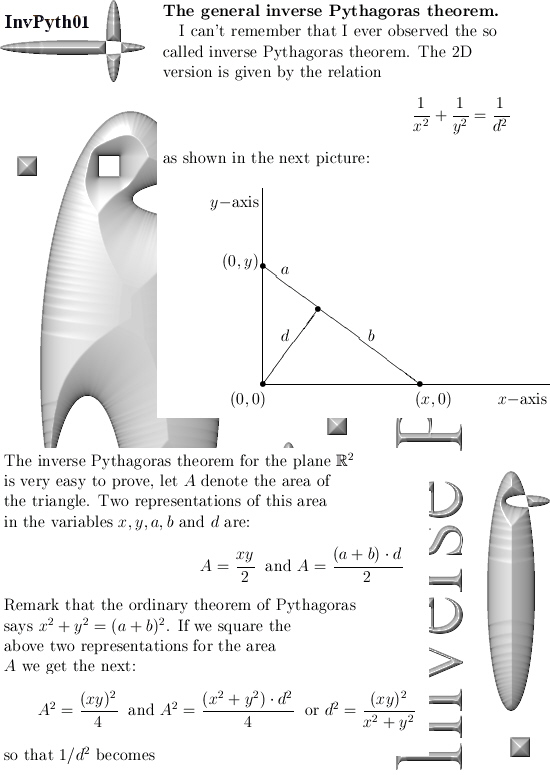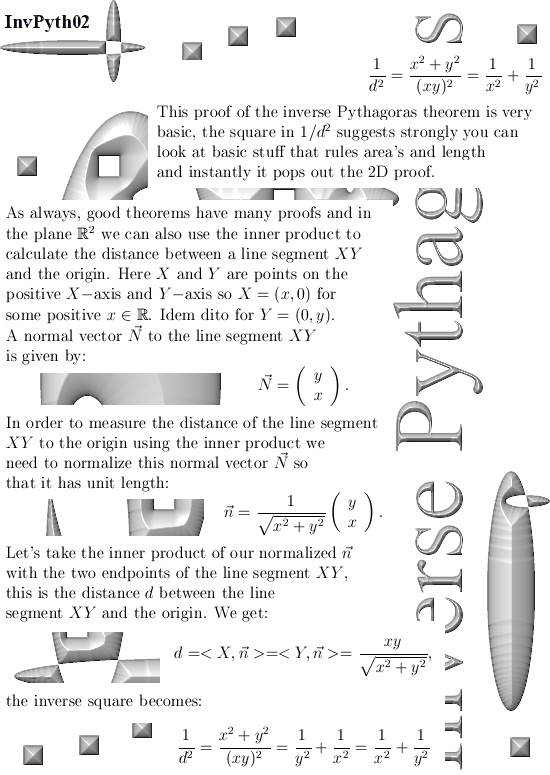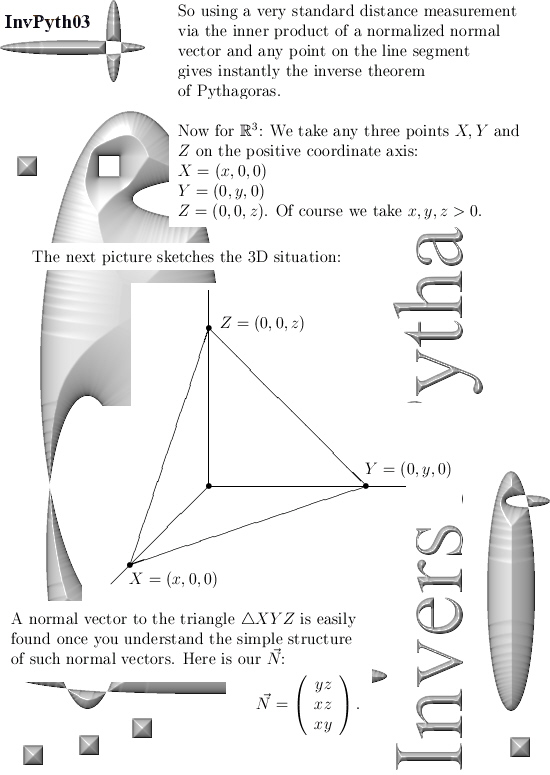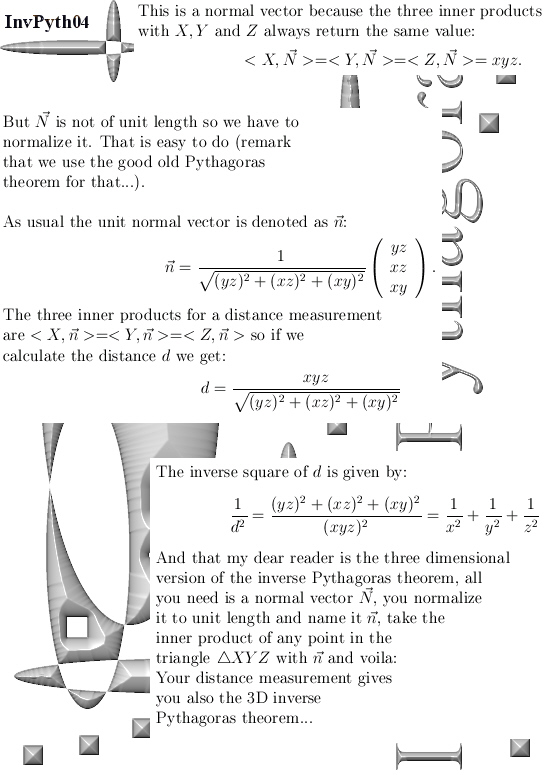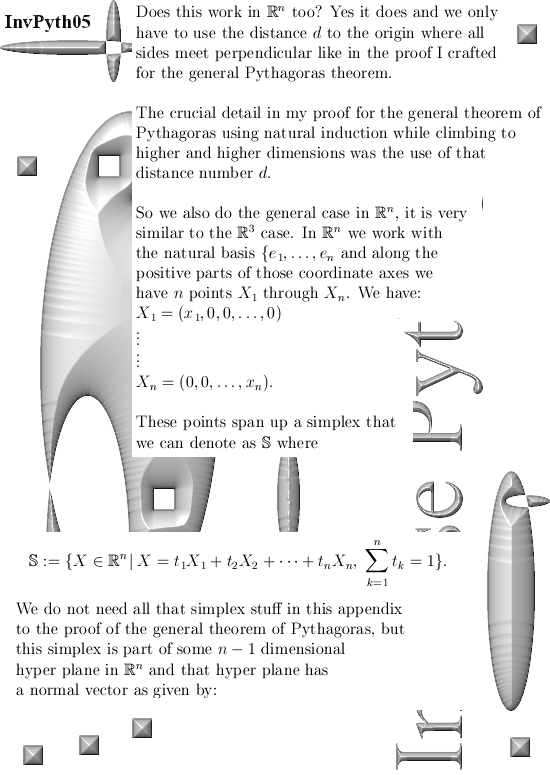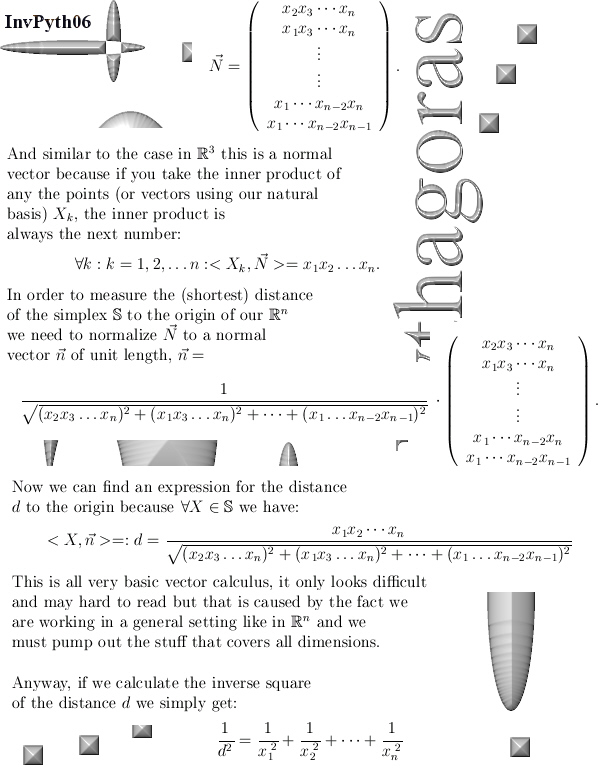That is a cute result but for me the normal vector is just as cute but only a bit harder to write out because that part deals with general setting where the dimension n is not fixed.

For the time being is this the end of this post. See you around my dear reader.

__________

Addendum added on 30 March 2018: In the previous post I forgot to place a link to the proof of the general theorem of Pythagoras as I crafted it once a long time ago.

Before this link I would like to show you once more how to prove the general theorem of Pythagoras for the 3D case using only the 2D theorem.

After all, that is the first basic step in my proof for the general theorem of Pythagoras…

Here are the two addendum pictures outlining how this basic step from the two dimensional plane to the 3D space goes: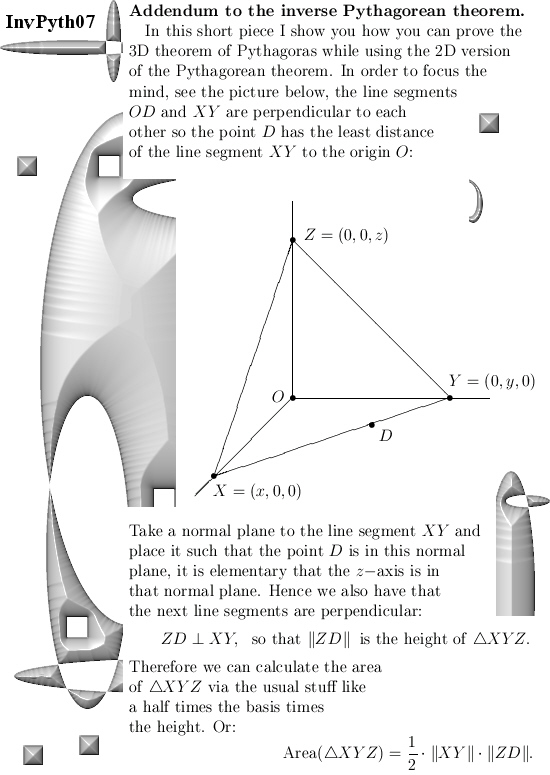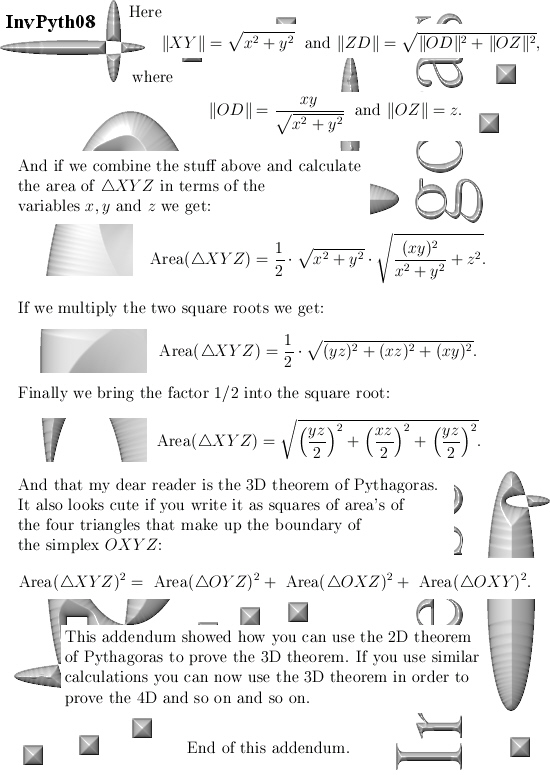Here is the link to the proof of the general theorem of Pythagoras:

The general theorem of Pythagoras (second and final post).

The general theorem of Pythagoras (second and final post).

Ok that was it, till updates.